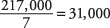## Measures of Variability

Measures of central tendency locate only the center of a distribution of measures. Other measures often are needed to describe data.

For example, consider the two sets of numbers presented in Table 1.The mean, the median, and the mode of each employee's daily earnings all equal \$200. Yet, there is significant difference between the two sets of numbers. For example, the daily earnings of Employee A are much more consistent than those of Employee B, which show great variation. This example illustrates the need for measures of variation or spread.

#### Range

The most elementary measure of variation is range. Range is defined as the difference between the largest and smallest values. The range is a single number. To say that the range is from \$190 to \$200, although informative, is not really a correct use of the term. The range for Employee A is \$210 – \$190 = \$20; the range for Employee B is \$400 – \$0 = \$400.

#### Deviation and variance

The deviation is defined as the distance of the measurements away from the mean. In Table 1, Employee A's earnings have considerably less deviation than Employee B's do. The variance is defined as the sum of the squared deviations of n measurements from their mean divided by ( n – 1).

So, from Table 1, the mean for Employee A is \$200, and the deviations from the mean are as follows:

0, +10, –10, +1, –1, –5, +5, 0

The squared deviations from the mean, therefore, are the following:

0, 100, 100, 1, 1, 25, 25, 0

The sum of these squared deviations from the mean equals 252. Dividing by ( n – 1), or 8 – 1, yields. So, the variance is 36.

For Employee B, the mean is also \$200, and the deviations from the mean are as follows:

0, –180, +200, –200, +190, –190, 0, +180

The squared deviations, therefore, are the following:

0; 32,400; 40,000; 40,000; 36,100; 36,100; 0; 32,400

The sum of these squared deviations equals 217,000. Dividing by ( n – 1) yields.

Although they earned the same totals, there is significant difference in variance between the daily earnings of the two employees.

#### Standard deviation

The standard deviation is defined as the positive square root of the variance; thus, the standard deviation of Employee A's daily earnings is the positive square root of 36, or 6. The standard deviation of Employee B's daily earnings is the positive square root of 31,000, or about 176.

#### Notation

s 2 denotes the variance of a sample.

σ 2 denotes the variance of a population.

s denotes the standard deviation of a sample.

σ denotes the standard deviation of a population.

#### Empirical rule: The normal curve

One practical significance of the standard deviation is that, with mound‐shaped (bell‐shaped) distributions, the following rules apply:
• The interval from one standard deviation below the mean to one standard deviation above the mean contains approximately 68 percent of the measurements.

• The interval from two standard deviations below the mean to two standard deviations above the mean contains approximately 95 percent of the measurements.

• The interval from three standard deviations below the mean to three standard deviations above the mean contains approximately all the measurements.

These mound‐shaped curves usually are called normal distributions or normal curves (see Figures 1, 2, and 3).

Figure 1.The interval ±σ from the mean contains 68 percent of the measurements.